Courses

# Solutions of Reflection of Light (Page No- 189) - Physics By Lakhmir Singh, Class 10 Class 10 Notes | EduRev

## Class 10 Physics Solutions By Lakhmir Singh & Manjit Kaur

Created by: Ajay Batra

## Class 10 : Solutions of Reflection of Light (Page No- 189) - Physics By Lakhmir Singh, Class 10 Class 10 Notes | EduRev

The document Solutions of Reflection of Light (Page No- 189) - Physics By Lakhmir Singh, Class 10 Class 10 Notes | EduRev is a part of the Class 10 Course Class 10 Physics Solutions By Lakhmir Singh & Manjit Kaur.
All you need of Class 10 at this link: Class 10

Lakhmir Singh Physics Class 10 Solutions Page No:189

Question 1: For what position of an object, a concave mirror forms a real image equal in size to the object ?

Solution : At the centre of curvature.

Question 2: Where should an object be placed in front of the concave mirror so as to obtain its virtual, erect and magnified image ?

Solution : Between pole and focus.

Question 3: For which positions of the object does a concave mirror produce an inverted, magnified and real image ?

Solution : Between focus and centre of curvature.

Question 4: If an object is placed at the focus of a concave mirror, where is the image formed ?

Solution : At infinity.

Question 5: If an object is at infinity (very large distance) in front of a concave mirror, where is the image formed ?

Solution : At focus.

Question 6: For what position of an object, a real and diminished image is formed by a concave mirror ?

Solution : Beyond the centre of curvature.

Question 7: Copy this figure in your answer book and show the direction of the light ray after reflection :Solution :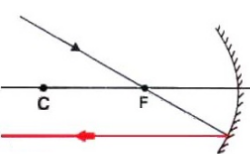Direction of light fay after reflection

Question 8: Draw the following diagram in your answer book and show the formation of image of the object AB with the help of suitable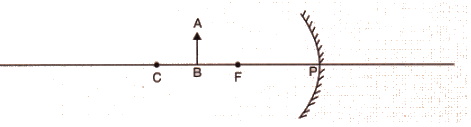Solution :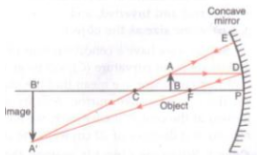Question 9: Draw the following diagram in your answer book and show the formation of image with the help of suitable rays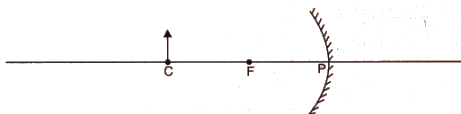Solution :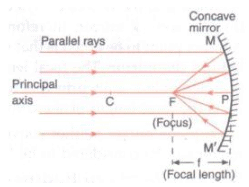Fig. formation of image of AC

Question 10: Which type of mirror could be used as a dentist’s mirror ?

Solution : Concave mirror.

Question 11: Which kind of mirror is used in the headlights of a car ? Why is it used for this purpose ?

Solution : Concave mirror is used in the headlights of a car. This is because when a lighted bulb is placed at the focus of a concave mirror reflector, then the diverging light rays of the bulb are collected by the concave reflector and then reflected to produce a strong, parallel-sided beam of light (which travels a considerable distance in the darkness of night).

Question 12: Explain why, a ray of light passing through the centre of curvature of a concave mirror gets reflected back along the same path.
Solution : A ray of light passing through the centre of curvature of a concave mirror is reflected back along the same path because it strikes the concave mirror at right angles to its surface due to which the angle of incidence and angle of reflection both are oo.

Question 13: What is the minimum number of rays required for locating the image formed by a concave mirror for an object ? Draw a ray diagram to show the formation of a virtual image by a concave mirror.

Solution : Minimum two rays are required for locating the image formed by a concave mirror for an object.
Ray diagram for the formation of a virtual image by a concave mirror:Question 14: With the help of a ray diagram, determine the position, nature and size of the image formed of an object
placed at the centre of curvature of a concave mirror.

Solution : When an object is placed at the centre of curvature (C) of a concave mirror, the image formed is:
(i) at the centre of curvature (C),
(ii) real and inverted,
(iii) of same size as the object.

Question 15: Describe with the help of a diagram, the nature, size and position of the image formed when an object is placed beyond the centre of curvature of a concave mirror.

Solution : When an object is placed beyond the centre of curvature (C) of a concave mirror, the image is:
(i) between the focus and centre of curvature,
(ii) real and inverted,
(iii) smaller than the object (or diminished).

Question 16: If an object is placed at a distance of 8 cm from a concave mirror of focal length 10 cm, discuss the nature of the image formed by drawing the ray diagram.

Solution : The focal length of the mirror is PF = 10 cm.
The object is placed at B such that PB = 8 cm. This means that the object lies between the pole and focus of the concave mirror.
The image formed is virtual, erect and magnified.

Question 17: Draw a ray diagram showing how a concave mirror can be used to produce a real, inverted and diminished image of an object.

Solution :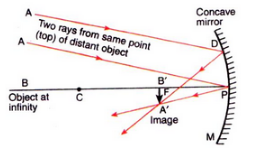Offer running on EduRev: Apply code STAYHOME200 to get INR 200 off on our premium plan EduRev Infinity!

94 docs

,

,

,

,

,

,

,

,

,

,

,

,

,

,

,

,

,

,

,

,

,

,

,

,

;